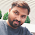### Problem

Given an array of n positive integers and a positive integer s, find the minimal length of a subarray of which the sum ≥ s. If there isn't one, return 0 instead.

### Example

Given the array [2,3,1,2,4,3] and s = 7, the subarray [4,3] has the minimal length of 2 under the problem constraint.

### JavaScript Code

```function minSubArrayLen(s, nums) {
if(nums == null || nums.length == 0){
return 0;
}

// initialize min length to be the input array length
var result = nums.length;

var i = 0;
var sum = nums;

for(var j=0; j<nums.length; ){
if(i==j){
if(nums[i]>=s){
return 1; //if single elem is large enough
}else{
j++;

if(j<nums.length){
sum = sum + nums[j];
}else{
return result;
}
}
}else{
//if sum is large enough, move left cursor
if(sum >= s){
result = Math.min(j-i+1, result);
sum = sum - nums[i];
i++;
//if sum is not large enough, move right cursor
}else{
j++;

if(j<nums.length){
sum = sum + nums[j];
}else{
if(i==0){
return 0;
}else{
return result;
}
}
}
}
}

return result;
}

console.log(minSubArrayLen(7, [2,3,1,2,4,3]));
```

1.this my another solution. first you have to sort thee array and then return with the specify position which you want.
var arr=[3,2,1,5,6,4];
function nthHighest(arr, n) {
var sorted = arr.sort(function (a, b) {
return a - b;
});
return sorted[sorted.length - n];
}
console.log(nthHighest(arr,n));

2.Thanks for sharing.I found a lot of interesting information here. A really good post, very thankful and hopeful that you will write many more posts like this one.
ViaMichelin
messenger
kodi.software/

3.Thanks a lot for sharing. Will check back later for more of your articles. penghilang bekas jerawat

## BlogrollSrinivas Dasari find me on facebook follow me on twitter